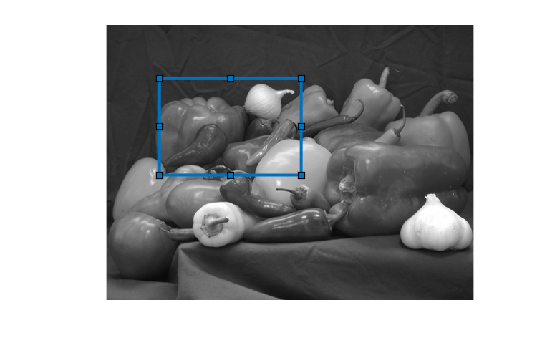# normxcorr2

Normalized 2-D cross-correlation

## Syntax

``C = normxcorr2(template,A)``

## Description

example

````C = normxcorr2(template,A)` computes the normalized cross-correlation of the matrices `template` and `A`. The resulting matrix `C` contains the correlation coefficients.```

## Examples

collapse all

Read two images into the workspace, and convert them to grayscale for use with `normxcorr2`. Display the images side-by-side.

```onion = im2gray(imread('onion.png')); peppers = im2gray(imread('peppers.png')); montage({peppers,onion})```Perform cross-correlation, and display the result as a surface.

```c = normxcorr2(onion,peppers); surf(c) shading flat```Find the peak in cross-correlation.

`[ypeak,xpeak] = find(c==max(c(:)));`

Account for the padding that `normxcorr2` adds.

```yoffSet = ypeak-size(onion,1); xoffSet = xpeak-size(onion,2);```

Display the matched area by using the `drawrectangle` function. The 'Position' name-value pair argument specifies the upper left coordinate, width, and height of the ROI as the 4-element vector `[xmin,ymin,width,height]`. Specify the face of the ROI as fully transparent.

```imshow(peppers) drawrectangle(gca,'Position',[xoffSet,yoffSet,size(onion,2),size(onion,1)], ... 'FaceAlpha',0);```## Input Arguments

collapse all

Input template, specified as a numeric matrix. The values of `template` cannot all be the same.

Data Types: `single` | `double` | `int8` | `int16` | `int32` | `int64` | `uint8` | `uint16` | `uint32` | `uint64` | `logical`

Input image, specified as a numeric image. `A` must be larger than the matrix `template` for the normalization to be meaningful.

Normalized cross-correlation is an undefined operation in regions where `A` has zero variance over the full extent of the template. In these regions, `normxcorr2` assigns correlation coefficients of zero to the output `C`.

Data Types: `single` | `double` | `int8` | `int16` | `int32` | `int64` | `uint8` | `uint16` | `uint32` | `uint64` | `logical`

## Output Arguments

collapse all

Correlation coefficients, returned as a numeric matrix with values in the range [-1, 1].

Data Types: `double`

## Algorithms

`normxcorr2` uses the following general procedure , :

1. Calculate cross-correlation in the spatial or the frequency domain, depending on size of images.

2. Calculate local sums by precomputing running sums.

3. Use local sums to normalize the cross-correlation to get correlation coefficients.

The implementation closely follows the formula from :

`$\gamma \left(u,v\right)=\frac{{\sum }_{x,y}\left[f\left(x,y\right)-{\overline{f}}_{u,v}\right]\left[t\left(x-u,y-v\right)-\overline{t}\right]}{{\left\{{{\sum }_{x,y}\left[f\left(x,y\right)-{\overline{f}}_{u,v}\right]}^{2}{\sum }_{x,y}{\left[t\left(x-u,y-v\right)-\overline{t}\right]}^{2}\right\}}^{0.5}}$`

where

• $f$ is the image.

• $\overline{t}$ is the mean of the template

• ${\overline{f}}_{u,v}$ is the mean of $f\left(x,y\right)$ in the region under the template.

 Lewis, J. P. "Fast Normalized Cross-Correlation." Industrial Light & Magic, 1995. http://scribblethink.org/Work/nvisionInterface/nip.pdf.

 Haralick, Robert M., and Linda G. Shapiro, Computer and Robot Vision, Volume II, Addison-Wesley, 1992, pp. 316-317.